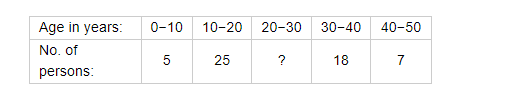# Calculate the missing frequency from the following distribution,

Question:

Calculate the missing frequency from the following distribution, it being given that the median of the distribution is 24.Solution:

Let the frequency of the class $20-30$ be $f_{1}$. It is given that median is 35 which lies in the class $20-30$. So $20-30$ is the median class.

Now, lower limit of median class $(l)=20$

Height of the class $(h)=10$

Frequency of median class $(f)=f_{1}$

Cumulative frequency of preceding median class $(F)=5+25$

Total frequency $(N)=55+f_{1}$

Formula to be used to calculate median,

$=l+\left(\frac{\frac{N}{2}-F}{f}\right)(h)$

Where,

I - Lower limit of median class

$h$-Height of the class

$f$ - Frequency of median class

$F$ - Cumulative frequency of preceding median class

$N$ - Total frequency

Put the values in the above,

$24=20+\left(\frac{\frac{\left(55+f_{1}\right)}{2}-30}{f_{1}}\right)(10)$

$\frac{4}{10}=\frac{55+f_{1}-60}{2 f_{1}}$

$2 f_{1}=50$

$f_{1}=25$

Hence, the required frequency is 25.